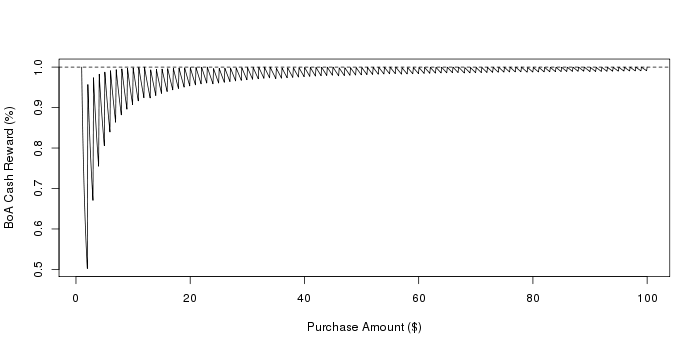# Bank of America 1% Cash Rewards Aren't Really 1%

Bank of America (BoA) has a "Cash Rewards" credit card that pays "1% cash back everywhere, every time"1. But if you read the fine print, it's clear that the reward is almost always less than 1%. Here's the relevant sentence from the terms and conditions2:

Fractions are truncated at the 100th decimal place, and are subject to verification...

This sentence is cryptic, and the context only helps a little bit. It means that for each purchase amount m, the reward is the value of m * 0.01 truncated at the 100th decimal place. For example, suppose that m = \$10.59. One percent of m is \$0.1059. The reward is then \$0.10.

This means that rewards are not paid on the fractional part of purchase amounts; that the full 1% is paid only for whole dollar amounts. Otherwise, the reward is less than 1%. As evidenced by my own transaction history, fractional purchase amounts are common. Hence, the full 1% cash reward is almost never achieved.

The actual cash reward percentage that BoA pays on each purchase depends on (1) the fractional purchase amount, and (2) the total purchase amount. For factional dollar amounts, the reward approaches 1% as the total amount becomes larger. And again, for whole dollar amounts, the percentage is exactly 1%. As a function of purchase amount, the cash reward percentage has a "saw tooth" shape:

```x <- seq(1.00, 100, length.out=1000)
plot(x, trunc(x)/x, type="l", xlab="Purchase Amount (\$)", ylab="BoA Cash Reward (%)")
abline(h=1, lty=2)
```Consumers who tend to make small purchases will generally receive a smaller cash reward percentage than those who make larger purchases. To illustrate, consider spending \$1000 dollars in 50 small purchases versus spending all \$1000 in two large purchases. We can simulate these two strategies by drawing the proportions spent at each transaction from the Dirichlet distribution.

```simulate <- function() {
g50 <- rgamma(50, 2, 1)
m50 <- round(g50 / sum(g50) * 1000, 2)
r50 <- trunc(m50) * 0.01
g2  <- rgamma(2, 2, 1)
m2  <- round(g2 / sum(g2) * 1000, 2)
r2  <- trunc(m2) * 0.01
c(sum(r50),sum(r2))
}

intervals <- apply(replicate(1000, simulate()), 1,
quantile, probs=c(0.025, 0.5, 0.975))
```

In 95% of these simulations, the reward amount for the first strategy (50 small purchases) was between \$9.71 (0.971%) and \$9.79 (0.979%), and between \$9.99 (0.999%) and \$9.99 (0.999%) for the second strategy (2 large purchases).